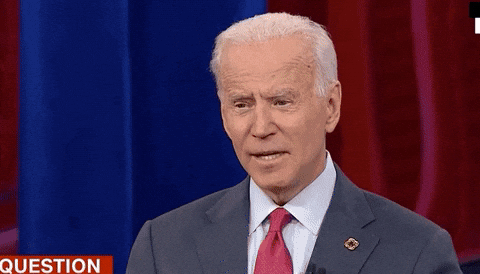## DEV Community 👩‍💻👨‍💻 is a community of 930,237 amazing developers

We're a place where coders share, stay up-to-date and grow their careers.# Convert Integer value to Hour:Minute:Seconds in dart

As a developer, there are times that you 'll need to convert a integer values to time remaining, It could be during the course of using Stream or something else.

Below is a good approach to handle the situation

Not so fast,To understand the code below you need to understand some basic time conversion.

## 1. one(1) hour =3600 seconds

Definitely to convert let's say 2 hours to seconds we 'll have 2*3600 = 7200 seconds

## 2. one(1) minute = 60 seconds

Definitely to convert 2minutes to seconds we 'll have
2*60 =120 seconds

## 3. one(1) seconds = 1 seconds

``````String intToTimeLeft(int value) {
int h, m, s;

h = value ~/ 3600;

m = ((value - h * 3600)) ~/ 60;

s = value - (h * 3600) - (m * 60);

String result = "\$h:\$m:\$s";

return result;
}
``````

Output

``````
0:0:0
``````

## And if you decide to go with one with more muscle

``````String intToTimeLeft(int value) {
int h, m, s;

h = value ~/ 3600;

m = ((value - h * 3600)) ~/ 60;

s = value - (h * 3600) - (m * 60);

String hourLeft = h.toString().length < 2 ? "0" + h.toString() : h.toString();

String minuteLeft =
m.toString().length < 2 ? "0" + m.toString() : m.toString();

String secondsLeft =
s.toString().length < 2 ? "0" + s.toString() : s.toString();

String result = "\$hourLeft:\$minuteLeft:\$secondsLeft";

return result;
}
``````

Output

``````
00:00:00
``````

That wraps up everything ..See you in the next one

## Top comments (2)Pablo Discobar

Good job! If you are interested in this topic, you can also look at my article about free vs paid Flutter templates. I'm sure you'll find something useful there, too.  - dev.to/pablonax/free-vs-paid-flutt...

#### Head to your account's Settings to...

🌚 Enable dark mode
🔠 Change your default font
📚 Adjust your experience level to see more relevant content